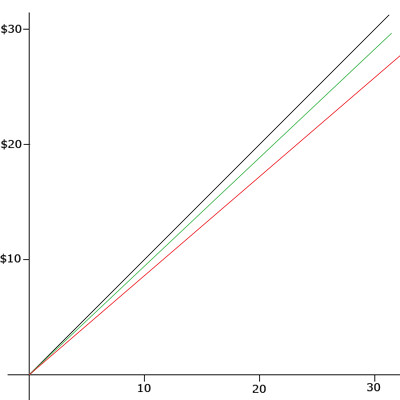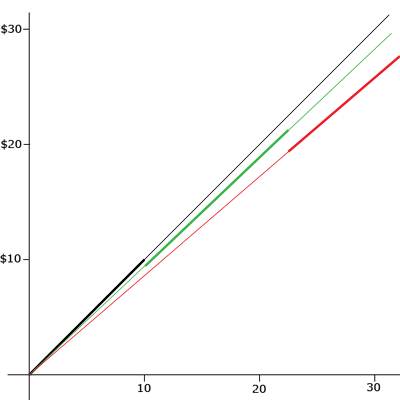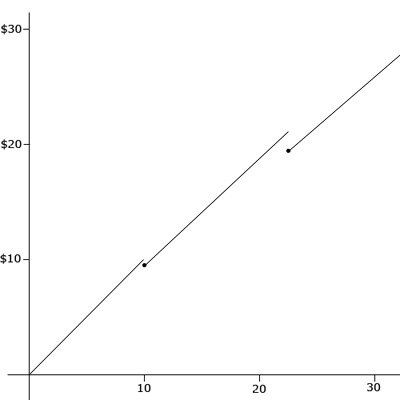Subject: piecewise functions
Name: Claudia
Who are you: Student (Secondary)

hi! i was just looking at a question by someone else about piecewise functions, but i still don't get it.
my problem is
g(x){x+2 if x <-2}
g(x){2x - 1 if x> or = -2}
thanks
-Claudia

Hi Claudia,

You didn't say what you were to do with the function g(x). Are you to graph it?

Yesterday I was looking in a catalogue for the cost of some fasteners I need and the catalogue entry is

1+ 10+ 25+
30 mm Square Latch \$1.00 \$0.85 \$0.75

This is a piecewise function but described in a table form rather than using functional notation. Suppose that n is the number of fasteners I want to buy and c(n) is the cost then in functional notation this function is

c(n) = \$1.00n if n is between 0 and 9
c(n) = \$0.85n if n is between 10 and 24
c(n) = \$0.75n if n is 25 or more

or using inequalities

c(n) = \$1.00n if 0 ≤ n < 10
c(n) = \$0.85n if 10 ≤ n < 25
c(n) = \$0.75n if 25 ≤ n

I plotted all three functions on the same graphc(n) = n, c(n) = 0.85 n, c(n) = 0.75 n

But you want c(n) = n for n less than 10, c(n) = 0.85 n for n between 10 and 25 and c(n) = 0.75 n for n larger than 25.Here is my final graph.I put two dots on the graph at the break points. This is just to clarify the value at these points. For example if you purchase 10 fasteners it's not clear from the graph without the extra point whether you pay \$10.00 or \$8.50.

I hope this helps,

Penny